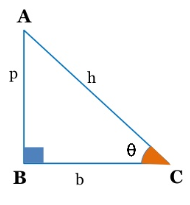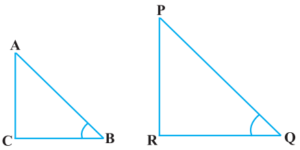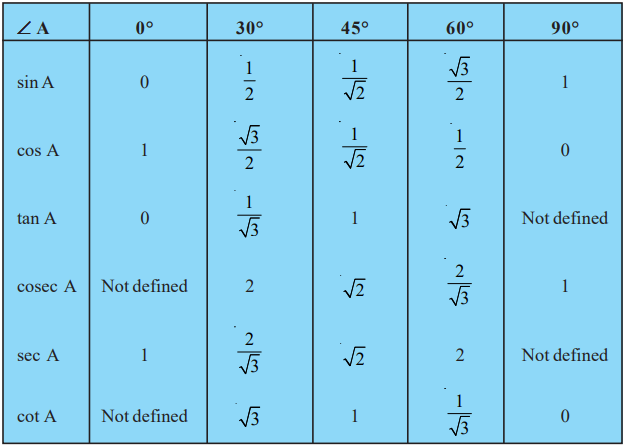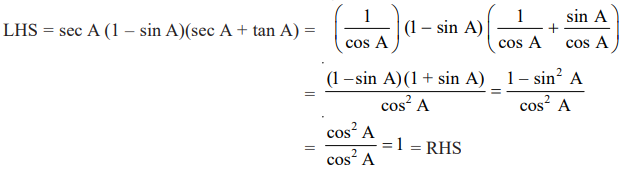Friday, October 22, 2021
Home > CBSE Class 10 > Trigonometry CBSE NCERT Notes Class 10 Maths Chapter 8 PDF

# Trigonometry CBSE NCERT Notes Class 10 Maths Chapter 8 PDFHi students, Welcome to AMBiPi (Amans Maths Blogs). In this article, you will get Introduction to Trigonometry CBSE NCERT Notes Class 10 Maths Chapter 8 PDF. You can download this PDF and save it in your mobile device or laptop etc.

# Introduction to Trigonometry CBSE NCERT Notes Class 10 Maths Chapter 8 PDF

## Trigonometry

Trigonometry is the study of relationships between the sides and angles of a triangle.

Read More : Real Life Application of Trigonometry

To convert an angle in degree into radian, we need to multiply π/180 to the degree.

To convert an angle in radian into degree, we need to multiply 180/π to the degree.

### Class 10 Maths Chapter 8 Examples 1:

30 degree = 30 x π/180 = π/6 radian.

### Class 10 Maths Chapter 8 Examples 2:

5π/12 radian = 5π/12 x 180/π = 75 degree.

## Arc Length of Sector of Circle

If an arc makes an angle θ (in radian) at the center of a circle whose radius is r, then the length of an arc of the arc is s = rθ. Hence, we can say that the angle θ = s/r radian.## Pythagoras Theorem

In a right angled triangle, the sum of the square of its perpendicular and the square of its base is equal to the square of the hypotenuse. This is known as Pythagorean or Pythagoras theorem.

Thus, in a right triangle, according to the Pythagorean theorem p2 + b2 = h2.## Trigonometric Ratios

In trigonometry, the trigonometric ratios of an acute angle in a right triangle express the relationship between the angle and the length of its sides.

The values of the trigonometric ratios of an angle do not vary with the lengths of the sides of the triangle, if the angle remains the same.1. Sine of θ is denoted as sinθ and it is the ratio of perpendicular (p) and hypotenuse (h). It means,

sinθ = p/h = AB/AC.

2. Cosine of θ is denoted as cosθ and it is the ratio of base (b) and hypotenuse (h). It means,

cosθ = b/h = BC/AC.

3. Tangent of θ is denoted as tanθ and it is the ratio of perpendicular (p) and base (b). It means,

tanθ = p/b = AB/BC.

4. Cosecant of θ is denoted as cosecθ or cscθ and it is the ratio of hypotenuse (h) and perpendicular (p). It means,

cosecθ or cscθ = h/p = AC/AB.

5. Secant of θ is denoted as secθ and it is the ratio of hypotenuse (h) and base (b). It means,

secθ = h/b = AC/BC.

6. Cotangent of θ is denoted as cotθ and it is the ratio of base (b) and hypotenuse (h). It means,

cotθ = b/p = BC/AB.

## Important Result of Trigonometric Ratios

There are following results about the trigonometric ratios.

7. Since sinθ = p/h, cosθ = b/h and tanθ = p/b, then tanθ = (p/h) / (b/h). Thus,

tanθ = sinθ / cosθ.

8. Since sinθ = p/h, cosθ = b/h and cotθ = b/p, then cotθ = (b/h) / (p/h). Thus,

cotθ = cosθ / sinθ.

9. Since sinθ = p/h and cosecθ = h/p, then

sinθ x cosecθ = 1 or sinθ = 1/ cosecθ and cosecθ = 1/ sinθ.

10. Since cosθ = b/h and secθ = h/b, then

cosθ x secθ = 1 or cosθ = 1/ secθ and secθ = 1/ cosθ.

11. Since tanθ = p/b and cotθ = b/p, then

tanθ x cotθ = 1 or tanθ = 1/ cotθ and cotθ = 1/ tanθ.

### Class 10 Maths Chapter 8 Examples 3:

Given that tan A = 4/3 , find the other trigonometric ratios of the angle A.Since tanA = 4/3 = BC/AB, then let AB = 3k and BC = 4k.

Using Pythagoras theorem, we get AC = 5k.

Thus,

sinA = BC/AC = 4/5,

cosA = AB/AC = 3/5,

cosecA = 1/sinA = 5/4,

secA = 1/cosA = 5/3,

cotA = 1/tanA = 3/4

### Class 10 Maths Chapter 8 Examples 4:

If ∠ B and ∠ Q are acute angles such that sin B = sin Q, then prove that ∠ B = ∠ Q.

Let two right triangles ABC and PQR as below, where sin B = sin Q.Given that sin B = sin Q ⇒ AC/AB = PR/PQ = k. Then, NowNow, AC/AB = PR/PQ = BC/QR.

Thus, by SSS Similarity, we get Δ ACB ~ Δ PRQ and therefore, ∠ B = ∠ Q.

## Trigonometric Ratios of Some Specific Angles### Class 10 Maths Chapter 8 Examples 5:

If sin (A – B) = 1/2, cos (A + B) = 1/2, 0° < A + B ≤ 90°, A > B, find A and B.

Since sin (A – B) = 1/2 ⇒ A – B = 30° and cos (A + B) = 1/2 ⇒ A + B = 60°

Solving these two equations, we get A = 45° and B = 15°.

## Trigonometric Ratios of Complementary Angles

The angles whose sum is 90 degree, then both are called complementary angles.

There are following trigonometric ratios for complementary angles.

sin(90o – θ) = +cosθ

cos(90o – θ) = +sinθ

tan(90o – θ) = +cotθ

cosec(90o – θ) = +secθ

sec(90o – θ) = +cosecθ

cot(90o – θ) = +tanθ

### Class 10 Maths Chapter 8 Examples 6:

If sin 3A = cos (A – 26°), where 3A is an acute angle, find the value of A.

sin 3A = cos (90° – 3A) ⇒ cos (90° – 3A) = cos (A – 26°) ⇒ 90° – 3A = A – 26° ⇒ A = 29°.

## Trigonometric Identities

Using Pythagorean theorem p2 + b2 = hand the basic definition of trigonometric ratios, we get three trigonometric identities as below.

sin2θ + cos2θ = 1

sec2θ – tan2θ = 1

cosec2θ – cot2θ = 1

### Class 10 Maths Chapter 8 Examples 7:

Prove that secA (1 – sin A)(sec A + tan A) = 1.Click below to get CBSE Class 10 Maths Chapter wise Revision Notes PDF.

Chapter NoChapter name
Chapter 1 :Real Numbers CBSE Class 10 Maths Revision Notes
Chapter 2 :Polynomials CBSE Class 10 Maths Revision Notes
Chapter 3 :Pair of Linear Equations in Two Variables CBSE Class 10 Maths Revision Notes
Chapter 4 :Quadratic Equations CBSE Class 10 Maths Revision Notes
Chapter 5 :Arithmetic Progression CBSE Class 10 Maths Revision Notes
Chapter 6 :Triangles CBSE Class 10 Maths Revision Notes
Chapter 7 :Coordinate Geometry CBSE Class 10 Maths Revision Notes
Chapter 8 :Introduction to Trigonometry CBSE Class 10 Maths Revision Notes
Chapter 9 :Some Applications of Trigonometry CBSE Class 10 Maths Revision Notes
Chapter 10 :Circles CBSE Class 10 Maths Revision Notes
Chapter 11 :Constructions CBSE Class 10 Maths Revision Notes
Chapter 12 :Area Related to Circles CBSE Class 10 Maths Revision Notes
Chapter 13 :Surface Areas & Volumes CBSE Class 10 Maths Revision Notes
Chapter 14 :Statistics CBSE Class 10 Maths Revision Notes
Chapter 15 :Probability CBSE Class 10 Maths Revision Notes
AMBiPi
error: Content is protected !!